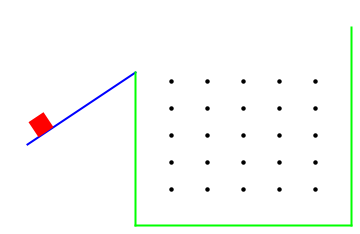Northwestern University: Machine Dynamics

### Technical Skills

• Lagrangian Dynamics
• Mathematica
• Rigid-body Motion
• External Forces
• Constrained Dynamics
• Impact Constraints

### Short Summary

The objective of this project was to create a system using Euler-Lagrange equations that has more than two degrees of freedom, contains rotational inertia, includes impacts, and has external forces. The game (modeled after the Plinko game from The Price is Right show) fulfills these requirements as an external force pushes the red square prism up a ramp against gravity. The force stops at the end of the ramp and the prism begins to freefall. As it does, it ricochets off the black pegs along with the green walls and floors. This causes it not only to translate in the plane but rotate as well. The equations describing the motion can be seen below.

Configuration Variables:$$\quad q = \{x(t),y(t),\theta(t)\}$$

Rotational Inertia:$$\quad I = \frac{8}{3}h\ell w(\ell^2+w^2) \qquad$$ Ramp Constraint: $$\quad y(t) - \frac{2}{3}x(t)-3$$

Kinetic Energy = $$\frac{1}{2}m(\dot x^2+\dot y^2)+\frac{1}{2}I\dot\theta^2 \qquad \qquad$$ Potential Energy = $$mgy(t) \qquad \qquad$$ Lagrangian = $$KE-PE$$

#### Pre-launch E-L Equations

• $$\frac{d}{dt}(\frac{\partial}{\partial \dot x}) - \frac{\partial L}{\partial x} = \lambda \frac{\partial \phi}{\partial x} + F \cos \theta$$

• $$\frac{d}{dt}(\frac{\partial}{\partial \dot y}) - \frac{\partial L}{\partial y} = \lambda \frac{\partial \phi}{\partial y} + F \sin \theta$$

• $$\frac{d}{dt}(\frac{\partial}{\partial \dot \theta}) - \frac{\partial L}{\partial \theta} = \lambda \frac{\partial \phi}{\partial \theta}$$

#### Post-launch E-L Equations

• $$\frac{d}{dt}(\frac{\partial}{\partial \dot x}) - \frac{\partial L}{\partial x} = 0$$

• $$\frac{d}{dt}(\frac{\partial}{\partial \dot y}) - \frac{\partial L}{\partial y} = 0$$

• $$\frac{d}{dt}(\frac{\partial}{\partial \dot \theta}) - \frac{\partial L}{\partial \theta} =0$$

#### Impact Constraints

Peg: At any point in time, the position of the x & y coordinates of each peg with respect to the body frame (square prism) is: $$\phi_x = x_{peg} \cos (\theta(t)) + y_{peg} \sin (\theta(t)) - \cos (\theta(t)) x(t) - \sin (\theta(t)) y(t)$$ $$\phi_y = y_{peg} \cos (\theta(t)) - x_{peg} \sin (\theta(t)) + \sin (\theta(t)) x(t) - \cos (\theta(t)) y(t)$$ These equations were generated by multiplying the transformation matrix, $$T_{sb}^{-1}$$, by the position vector of the peg $$r_{peg}$$ and extracting the x and y parts of the resulting column vector. $$r_{peg} = \begin{bmatrix} x_{peg} & y_{peg} & 1 \end{bmatrix}^T \qquad T_{sb} = \begin{bmatrix} \cos \theta(t) & -\sin \theta(t) & x(t) \\ \sin \theta(t) & \cos \theta(t) & y(t) \\ 0 & 0 & 1 \end{bmatrix} \to Inverse \to$$ $$T_{sb}^{-1} = \begin{bmatrix} \cos \theta(t) & \sin \theta(t) & -\cos (\theta(t)) x(t) -\sin (\theta(t)) y(t)\\ -\sin (\theta(t)) & \cos (\theta(t)) & \sin (\theta(t)) x(t) - \cos (\theta(t)) y(t) \\ 0 & 0 & 1 \end{bmatrix}$$ $$r_{peg\;in\;body} = T_{sb}^{-1} \cdot r_{peg} = \begin{bmatrix} \phi_x & \phi_y & 1 \end{bmatrix}$$ In other words, when $$\phi_x$$ and $$\phi_y$$ equal zero, the centroid of the square prism would be completely overlapping the centroid of the peg. However, in order to evaluate the impact update laws (see below), the edges are what need to be detected. To account for this, both $$\phi_x$$ and $$\phi_y$$ are subtracted by half the width and length of the prism respectively along with the radius of the peg and set equal to zero.

Wall or Floor: To determine if the prism is in collision with the wall or floor, the four corner points are used. As an example, the top right corner point of the prism in the world frame can be found by: $$r_{topRight} = T_{sb} \cdot r_{topRightInB}\qquad where \qquad r_{topRightInB} = \begin{bmatrix} \frac{\ell}{2} & \frac{w}{2} & 1 \end{bmatrix}^T$$ This results in $$\frac{\ell}{2}\cos(\theta(t))-\frac{w}{2}\sin(\theta(t)) + x(t)$$ so when this equation equals the $$x$$ location of the wall or the $$y$$ location of the floor, the impact update law below is evaluated.

#### Impact Update Laws

• $$\frac{\partial L}{\partial \dot x}(\tau^+) - \frac{\partial L}{\partial \dot x}(\tau^-) = \lambda \frac{\partial \phi}{\partial x}$$

• $$\frac{\partial L}{\partial \dot y}(\tau^+) - \frac{\partial L}{\partial \dot y}(\tau^-) = \lambda \frac{\partial \phi}{\partial y}$$
• $$\frac{\partial L}{\partial \dot \theta}(\tau^+) - \frac{\partial L}{\partial \dot \theta}(\tau^-) = \lambda \frac{\partial \phi}{\partial \theta}$$

• $$H(\tau^+) - H(\tau^-) = 0$$

### Demo Video